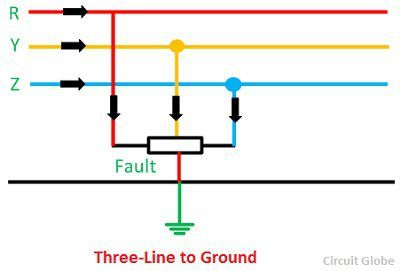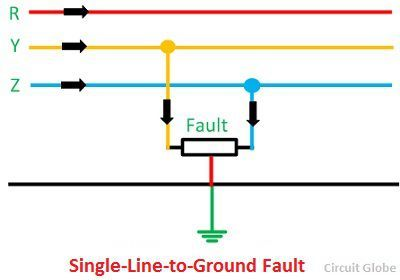# Types of Faults in Power System

The fault in the power system is defined as the defect in the power system due to which the current is distracted from the intended path. The fault creates the abnormal condition which reduces the insulation strength between the conductors. The reduction in insulation causes excessive damage to the system. The fault in the power system is mainly categorised into two types they are

1. Open Circuit Fault
2. Short Circuit Fault.

The different types of power system fault are shown below in the image.The faults in the power system may occur because of the number of natural disturbances like lightning, high-speed winds, earthquake, etc. It may also occur because of some accidents like falling off a tree, vehicle colliding, with supporting structure, aeroplane crashing, etc.

## 1. Open Circuit Fault

The open circuit fault mainly occurs because of the failure of one or two conductors. The open circuit fault takes place in series with the line, and because of this, it is also called the series fault. Such types of faults affect the reliability of the system. The open circuit fault is categorised  as

• Open Conductor Fault
• Two conductors Open Fault
• Three conductors Open Fault.

The open circuit fault is shown in the figure below.## 2. Short-Circuit Fault

In this type of fault, the conductors of the different phases come into contact with each other with a power line, power transformer or any other circuit element due to which the large current flow in one or two phases of the system. The short-circuit fault is divided into the symmetrical and unsymmetrical fault.

### Symmetrical Fault

The faults which involve all the three phases is known as the symmetrical fault. Such types of fault remain balanced even after the fault. The symmetrical faults mainly occur at the terminal of the generators. The fault on the system may arise on account of the resistance of the arc between the conductors or due to the lower footing resistance. The symmetrical fault is sub-categorized into line-to-line-to-line fault and three-phase line-to-ground-fault

a. Line – Line – Line Fault – Such types of faults are balanced, i.e., the system remains symmetrical even after the fault. The L – L – L fault occurs rarely, but it is the most severe type of fault which involves the largest current. This large current is used for determining the rating of the circuit breaker.b. L – L – L – G (Three-phase line to the ground fault) – The three-phase line to ground fault includes all the three phase of the system. The L – L – L – G fault occurs between the three phases and the ground of the system. The probability of occurrence of such type of fault is nearly 2 to 3 percent.### Unsymmetrical Fault

The fault gives rise to unsymmetrical current, i.e., current differing in magnitude and phases in the three phases of the power system are known as the unsymmetrical fault. It is also defined as the fault which involves the one or two phases such as L- G, L – L, L – L – G fault. The unsymmetrical makes the system unbalanced. It is mainly classified into three types. They are

1. Single Line-to-ground (L – G) Fault
2. Line-to-Line Fault (L – L)
3. Double Line-to-ground (L – L – G) Fault

The unsymmetrical fault is the most common types of fault occur in the power system.

1. Single Line-to-Line Ground – The single line of ground fault occurs when one conductor falls to the ground or contact the neutral conductor. The 70 – 80 percent of the fault in the power system is the single line-to-ground fault.2. Line – to – Line Fault  –  A line-to-line fault occurs when two conductors are short circuited. The major cause of this type of fault is the heavy wind. The heavy wind swinging the line conductors which may touch together and hence cause short-circuit. The percentage of such type of faults is approximately 15 – 20%.3. Double Line – to – line Ground Fault – In double line-to-ground fault, the two lines come in contact with each other along with the ground. The probability of such types of faults is nearly 10 %.The symmetrical and unsymmetrical fault mainly occurs in the terminal of the generator, and the open circuit and short circuit fault occur on the transmission line.